Skip to main content

# Application adaptive exponential synchronization of chaotic dynamical systems in secure communications

## Abstract

Synchronization of chaotic systems which is defined based on the exponential stability for encrypts of signals is presented in this paper. An adaptive control scheme is proposed, and the convergence of the synchronized error is guaranteed. Masking and modulation methods are applied for encryption. To verify the effectiveness of the proposed schemes, numerical simulation was applied on a well-known system without linear term chaotic using MatLab software. The comparison of the proposed chaotic synchronization in the case of the synchronization rate and in the decryption precision of sent signal and image are shown.

## Introduction

Chaotic systems are nonlinearly deterministic and sensitive to initial conditions, they have been studied since the two most recent decades . Chaotic systems have a lot of applications in different sciences such as laser, secure communication, biology sciences, nonlinear circuits, neural and computer networks .

The control of chaos is one of the main issues in the study of chaotic systems. The synchronization is one of the main control methods of these systems, which were introduced in a paper by Pecorra and Carrol in 1990 . In recent years, synchronization of chaotic systems has become very attractive and has been applied in vast area of engineering, physic, computer networks, and so on which aroused increasing interest as regards use in new areas such as cryptography .

Up to now, many synchronization methods such as adaptive control, nonlinear feedback control and sliding mode control were proposed and applied successfully on chaotic systems . But almost all of them used Lyapunov stability theorems to guarantee asymptotically synchronization and convergence is slow to the origin.

The exponential synchronization is another proposed method in this field, which has certain precision and stability in error systems. This method is robust and faster than the Lyapunov stability one. Tong et al.  applied exponential synchronization for stochastic neural networks with multi-delayed and Markovian switching via adaptive feedback control, Yan and Yu , Lio and Yu  studied exponential synchronization for some well-known classical dynamical systems. Yang used exponential stability in synchronization of a higher order chaotic system  etc.

So far, to the best of the authors’ knowledge, the problem of adaptive exponential synchronization and its applications for chaotic dynamical systems have received very little research attention.

In the recent work, the authors have proposed the adaptive based on exponential stability methods to secure chaos communication through the method of chaos masking and modulation. The general idea in the utilization of chaotic systems in using a transition of the desired signal is integration of it with a chaotic system and production of a chaotic signal. This signal is recoverable after synchronization. There are two major issues in this case; one includes how to produce the chaotic signal; this is usually conducted by both masking and modulation methods [24, 25].

The other most important issue is the time of transmittance and reception of the signal. The signal is produced and transmitted after synchronization of both slave and master systems. Thus, as the synchronization rate is high, the signal, with regard to its dependency on synchronization error, could be transmitted faster with better approximation.

This paper was conducted by introducing theorems related to the exponential stability and comparison of results in the encryption of signals based on Lyapunov stability and exponential stability.

The main contributions of this paper can be highlighted as follows. (1) A new adaptive synchronization method is studied for a fully nonlinear chaotic dynamical system based on the exponential stability theorem. (2) Application of the adaptive exponential synchronization obtained in encryption using modulation and masking methods.

The paper was organized as follows. Section 2, the definitions and theorems related to exponential and Lyapunov stability were provided. In Section 3, synchronization of a system without linear term is provided. Transmission and reception of the signal text by the modulation method was given for a well-known system using exponential and Lyapunov stability in Section 4. Then the masking method and adaptive exponential stability were utilized to transmit a pictorial signal. Concluding remarks were provided in Section 5.

## Preliminaries

### Stability of autonomous systems

Consider the autonomous system

$$\dot{x}=f(x),$$
(1)

where $$f: D\rightarrow R^{n}$$ is the local Lipschitz map of domain $$D\subset R^{n}$$ to $$R^{n}$$.

### Definition 2.1

Suppose $$x_{0}=0$$ is one of the fixed points of equation (1), in this case .

• It is stable if, for any ϵ, there exists the value of $$\delta=\delta(\epsilon)$$ so that

$$\big\| x(0)\big\| < \delta\quad\Rightarrow \quad\big\| x(t)\big\| < \epsilon, \quad \forall t>0.$$
• It is unstable if it is not stable.

• It is asymptotically stable, if it is stable and there exist δ so that

$$\big\| x(0)\big\| < \delta\quad\Rightarrow \quad\lim_{t\rightarrow\infty}x(t)=0.$$

For studying stability, a practical way is finding a Lyapunov function in linear and nonlinear systems without obtaining the response of them.

### Theorem 2.1

Lyapunov stability theorem

Suppose $$\dot{x}=f(x)$$, $$x\in D\subseteq R^{n}$$ to have a fixed point in the origin. If there exists a real function V in a neighborhood of N at the origin  where:

• $$\frac{\partial V}{\partial x_{i}}$$, $$i=1,2,\ldots,n$$, exists and is continuous;

• V is positive definite,

then:

• If is semi negative definite, then the origin is a stable fixed point of the system.

• If is negative definite, then the origin is a fixed point and asymptotically stable.

• If is positive definite, then the origin is an unstable fixed point.

### Stability of non-autonomous systems

Consider the following non-autonomous system:

$$\dot{x}=f(t,x),$$
(2)

where the function $$f: [0,\infty)\times D\rightarrow R^{n}$$ is piecewise continuous with respect to t and is local Lipschitz with respect to x on $$[0,\infty)\times D$$, which includes the origin. In this case $$x=0$$ is the fixed point of equation (2) on $$t=0$$ if

$$f(t,0)=0, \quad \forall t\geqslant0.$$

It could be interpreted as follows: the fixed point is located on the origin as a non-zero fixed point or in a more general case, as the non-zero response of system.

The concepts of stability and asymptotically stability of a fixed point in non-autonomous systems correspond to Definition 2.1 in autonomous systems unless there is a new case, in that the response of autonomous system only depends on $$(t-t_{0})$$, and the response of the non-autonomous system also depends on both t and $$t_{0}$$. Thus, in the general state, the stability of fixed point depends on $$t_{0}$$.

### Definition 2.2

Fixed point $$x=0$$ related to equation (2):

• It is stable if, for any $$\epsilon>0$$, the value of $$\delta =\delta(\epsilon,t_{0})$$ is such that

$$\big\| x(t_{0})\big\| < \delta\quad\Rightarrow\quad\big\| x(t)\big\| < \epsilon,\quad \forall t\geq t_{0}\geq0.$$
(3)
• It is uniformly stable if, for any $$\epsilon>0$$, the value of $$\delta=\delta(\epsilon)$$ (independent of $$t_{0}$$) is such that equation (3) holds.

• It is unstable, if is not stable.

• It is asymptotically stable if is stable and there is a value such as $$c=c(t_{0})>0$$ so that, for all values of $$\|x(t_{0})\|< c$$, we have $$\lim_{t\rightarrow\infty}x(t)=0$$.

• It is uniform asymptotically stable if it is uniformly stable and there is a value such as $$c>0$$ (independent of $$t_{0}$$) so that, for all values of $$\|x(t_{0})\|< c$$, as $$t_{0}$$ tends to infinity, $$x(t)$$ monotonically tends to zero with respect to $$t_{0}$$, in other words, for any $$\epsilon>0$$, there exists a value such as $$t=T(\epsilon)$$ so that

$$\big\| x(t)\big\| < \epsilon, \quad\forall t\geq t_{0}+T(\epsilon), \forall\big\| x(t_{0})\big\| < c.$$
• It is globally uniform asymptotically stable, if it is uniformly stable and, for any pair of positive numbers ϵ and c, there is a value $$t=T(\epsilon,c)>0$$ so that

$$\big\| x(t)\big\| < \epsilon, \quad \forall t\geq t_{0}+T(\epsilon,c), \forall\big\| x(t_{0})\big\| < c.$$

The uniform stability and uniform asymptotical stability could also be indicated based on specific numerical functions, i.e. class κ and class κι functions .

### Definition 2.3

The continuous function $$\alpha: [0, a)\rightarrow[0, \infty)$$ belongs to class κ if it strictly is increasing and $$\alpha (0)=0$$. This function belongs to $$\kappa_{\infty}$$ if $$a=\infty$$ and with r increasing to infinity, $$a(r)$$ also tends to infinity.

### Definition 2.4

The continuous function $$\beta: [0, a)\times[0, \infty)\rightarrow [0, \infty)$$ belongs to class κι if for any specific value s, the mapping $$\beta(r,s)$$ with respect to r belongs to class κ and also for any specific value r, the mapping $$\beta(r,s)$$ is decreasing with respect to s, and by increasing s to infinity, $$\beta(r,s)$$ tends to zero.

### Example 2.1

The function $$\alpha(r)=\arctan r$$ is increasing strictly because $$\dot {\alpha}(r)=1/(1+r^{2})>0$$. This function belongs to class κ, but it does not belong to class $$\kappa_{\infty}$$, because $$\lim_{r\rightarrow \infty}\alpha(r)=\pi/2<\infty$$.

The following lemma indicates some of explicit features of functions, class κ and κι.

### Lemma 2.2

Suppose $$\alpha_{1}(\cdot)$$ and $$\alpha_{2}(\cdot)$$ in the interval $$[0, a)$$ are class κ functions and $$\alpha_{3}(\cdot)$$ and $$\alpha_{4}(\cdot)$$ to be class $$\kappa_{\infty}$$ ones. If we show the converse of $$\alpha_{i}(\cdot)$$ with $$\alpha^{-1}_{i}(\cdot)$$, then we have 

• $$\alpha^{-1}_{1}(\cdot)$$ is defined in the interval $$[0, \alpha_{1}(a)]$$ belongs to class κ.

• $$\alpha^{-1}_{3}(\cdot)$$ is defined in the interval $$[0, \infty)$$ and belongs to class $$\kappa_{\infty}$$.

• $$\alpha_{1}o\alpha_{2}$$ belongs to class $$\kappa_{\infty}$$.

• $$\alpha_{3}o\alpha_{4}$$ belongs to class $$\kappa_{\infty}$$.

• $$\sigma(r, s)=\alpha_{1}(\beta(\alpha_{2}(r), s))$$ belongs to class $$\kappa_{\infty}$$.

The following lemma explains the equivalence definitions for uniform stability and uniformly asymptotically stability based on class κ and κι functions.

### Lemma 2.3



For the fixed point $$x=0$$ related to equation (2):

• It is uniformly stable, if and only if function $$\alpha(\cdot)$$ belongs to class κ and there is a positive constant c (independent of $$t_{0}$$) so that

$$\big\| x(t)\big\| \leq\alpha\bigl(\big\| x(t_{0})\big\| \bigr), \quad \forall t\geq t_{0}\geq0, \forall \big\| x(t_{0})\big\| < c.$$
(4)
• It is uniformly asymptotically stable, if and only if function $$\beta(\cdot, \cdot)$$ belongs to class κι and there is a positive constant c (independent of $$t_{0}$$) so that

$$\big\| x(t)\big\| \leq\beta\bigl(\big\| x(t_{0})\big\| \bigr), \quad \forall t \geq t_{0}\geq0, \forall\big\| x(t_{0})\big\| < c.$$
(5)
• It is globally uniform asymptotically stable if and only if inequality (5) holds for any initial state of $$x(t_{0})$$.

### Exponential stability

As one of Lemma 2.3 results, it could be considered that in the case of non-autonomous systems, the stability and asymptotical stability based on definition (1) mean that there are functions of class κ and κι so that inequality (4) and (5) hold. Because in the non-autonomous systems, the stability and asymptotically stability of origin are uniform with respect to the initial time $$t_{0}$$.

One particular case of uniform asymptotically stability occurs when, in equation (5), the function β belongs to class κι as $$\beta(r, s)=k r e^{-\gamma s}$$. This case is very important and could be considered one of distinctive features of fixed point stability.

### Definition 2.5

The fixed point $$x=0$$ related to equation (2) is exponentially stable, if inequality (5) holds for any of the following values:

$$\beta(r, s)=k r e^{-\gamma s}, \quad k>0, \gamma>0.$$

In addition, it is globally exponentially stable if the above condition holds for all initial values.

Now, we explain the main theorem.

### Theorem 2.4

Suppose $$x=0$$ to be one of the fixed points of equation (2) and $$D\subset R^{n}$$ to be a domain including the origin. In addition, suppose $$V(x): [0, \infty)\times D\rightarrow R$$ to be a continuous and derivable function where for all values of $$t\geq0$$ and $$x\in D$$ the following relations are satisfied:

\begin{aligned}& \omega_{1}(x)\leq V(t, x)\leq\omega_{2}(x), \end{aligned}
(6)
\begin{aligned}& \frac{\partial V}{\partial t}+\frac{\partial V}{\partial x}f(t, x)\leq-\omega_{3}(x), \end{aligned}
(7)

where $$\omega_{1}(x)$$, $$\omega_{2}(x)$$ and $$\omega_{3}(x)$$ are positive definite and continuous functions on D. Then the point $$x=0$$ is uniformly asymptotically stable .

If the class κ functions are of the particular form $$\alpha _{i}(r)=k_{i}r^{c}$$, its application is easy in the proof of Theorem 2.4. In this case, it could be shown that the origin is exponentially stable.

### Theorem 2.5

Suppose all hypotheses of Theorem 2.4 are satisfied along with the following conditions:

$$\omega_{1}(x)\geq k_{1}\|x\|^{c},\qquad \omega_{2}(x)\leq k_{2}\|x\|^{c}, \qquad\omega _{3}(x)\geq k_{3}\|x\|^{c},$$

when $$k_{1}$$, $$k_{2}$$, $$k_{3}$$ and c are positive constants, then $$x=0$$ is exponentially stable. Also, if all globally hypotheses hold, $$x=0$$ is globally exponentially stable .

### Proof

The functions V and satisfy the following inequalities:

\begin{aligned} &k_{1}\|x\|^{c}\leqq V(t, x)\leqq k_{2}\|x \|^{c}, \\ &\dot{V}\leqq-k_{3}\|x\|^{c}\leqq- \frac{k_{3}}{k_{2}}V(t, x). \end{aligned}
(8)

By using the deduction lemma, we could write

$$V\bigl(t, x(t)\bigr)\leqq V\bigl(t, x(t_{0})\bigr)e^{-(k_{3}/k_{2})(t-t_{0})}.$$

Therefore,

\begin{aligned} \big\| x(t)\big\| \leqq&\biggl[\frac{V(t, x(t))}{k_{1}}\biggr]^{1/c} \end{aligned}
(9)
\begin{aligned} \leqq& \biggl[\frac{V(t_{0}, x(t_{0}))e^{-(k_{3}/k_{2})(t-t_{0})}}{k_{1}}\biggr]^{1/c} \\ \leqq&\biggl[\frac{k_{2}\|x(t_{0})\|^{c}e^{-(k_{3}/k_{2})(t-t_{0})}}{k_{1}}\biggr]^{1/c} \\ =&\biggl(\frac{k_{2}}{k_{1}}\biggr)^{1/c}\big\| x(t_{0}) \big\| e^{-(k_{3}/k_{2}c)(t-t_{0})}. \end{aligned}
(10)

As a result, the origin is exponentially stable, so that all hypotheses are satisfied globally, the above inequality would hold for all values of $$x(t_{0})\in R^{n}$$. □

## Synchronization based on the exponential stability

In this section, the synchronization system without linear term is introduced based on exponential and Lyapunov stability using nonlinear control functions.

Consider the nonlinear chaotic system 

\begin{aligned} &\dot{x_{1}}=\ln\bigl(a+e^{y_{1}-x_{1}}\bigr), \\ &\dot{y_{1}}=x_{1}z_{1}, \\ &\dot{z_{1}}=b-x_{1}y_{1}, \end{aligned}
(11)

where $$x_{1}$$, $$y_{1}$$ and $$z_{1}$$ are the state variables and a, b are real parameters. Figures 1 and 2 show the Lyapunov exponent and the chaotic behavior of the system (11) for $$a=0.1$$ and $$b=0.25$$.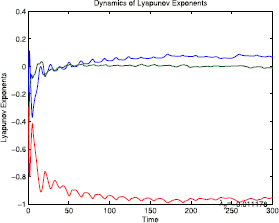Figure 1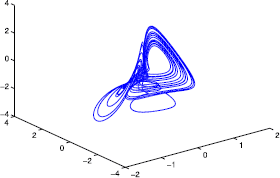Figure 2

For synchronization, let system (11) be the drive system and the corresponding response system be written as follows:

\begin{aligned} &\dot{x_{2}}=\ln\bigl(a+e^{y_{2}-x_{2}} \bigr)+u_{1}, \\ &\dot{y_{2}}=x_{2}z_{2}+u_{2}, \\ &\dot{z_{2}}=b-x_{2}y_{2}+u_{3}, \end{aligned}
(12)

where $$u_{1}$$, $$u_{2}$$ and $$u_{3}$$ are the input nonlinear control functions. By defining the synchronization error as $$e_{1}=x_{2}-x_{1}$$, $$e_{2}=y_{2}-y_{1}$$, $$e_{3}=z_{2}-z_{1}$$, and using equation (12), the synchronization error dynamics can be written as

\begin{aligned} &\dot{e_{1}}=\ln\biggl(\frac {a+e^{e_{2}-e_{1}}e^{y_{1}-x_{1}}}{a+e^{y_{1}-x_{1}}} \biggr)+u_{1}, \\ &\dot{e_{2}}=e_{1}e_{3}+e_{1}z_{1}+e_{3}x_{1}+u_{2}, \\ &\dot{e_{3}}=-(e_{2}e_{1}+y_{1}e_{1}+x_{1}e_{2})+u_{3}. \end{aligned}
(13)

### Definition 3.1

The response system (12) will synchronize exponentially with the drive system (11) for any initial values if the error system satisfies the following estimation:

$$E(t)^{T}E(t)\leq\alpha e^{-\sigma(t-t_{0})},$$
(14)

where $$E^{T}=[e_{1}(t),e_{2}(t),e_{3}(t)]$$ and α, σ are positive constants, respectively, dependent and independent from $$E(t_{0})$$, which is called the convergence rate. In this case the zero solution of error system (13) is stable and the two systems (11) and (12) are called synchronized .

### Lemma 3.1

The system (13) can be exponentially stabilized at the origin if there exists a positive definite function $$V(t)$$ such that

\begin{aligned}& k_{1}E^{T}(t)E(t)\leq V(t) \leq k_{2}E^{T}(t)E(t), \end{aligned}
(15)
\begin{aligned}& \dot{V(t)}\leq-k_{3}E^{T}(t)E(t), \end{aligned}
(16)

where $$k_{1}$$, $$k_{2}$$ and $$k_{3}$$ are positive constants, $$V(t)\equiv V(E(t))$$ and $$k_{1}\leq k_{2}$$. Therefore, the drive system (11) and the response system (12) are exponentially synchronized .

### Proof

Let $$V(t)=E(t)PE^{T}(t)$$ where P is a symmetric positive definite matrix. Choose $$k_{1}=\lambda_{\mathrm{min}}(P)>0$$ and $$k_{2}=\lambda_{\mathrm{max}}(p)>0$$ where $$k_{1}$$ and $$k_{2}$$ are minimum and maximum eigenvalues of the matrix P. Then the time derivative of $$V(t)$$ satisfies the inequality

$$\dot{V(t)}\leq-\frac{k_{3}}{k_{2}}V(t)=-\sigma V(t),\quad \sigma=\frac{k_{3}}{k_{2}}>0.$$
(17)

It can be concluded that

$$V(t)\leq V(t_{0})e^{\sigma(t-t_{0})}.$$
(18)

Hence

\begin{aligned} E^{T}(t)E(t)\leq\frac{V(t)}{k_{1}} \leq\frac{V(t_{0})}{k_{1}}e^{-\sigma (t-t_{0})} \leq\frac{k_{2}}{k_{1}}E^{T}(t_{0})E(t_{0})e^{-\sigma(t-t_{0})}. \end{aligned}
(19)

From Definition 3.1, $$\alpha=\frac {k_{2}}{k_{1}}E^{T}(t_{0})E(t_{0})$$. Therefore, in this case, system (13) is exponentially stable and the synchronization of the drive system (12) and the response system (11) sufficiently achieved. □

### Lemma 3.2

For any $$k\in R^{+}$$ and $$x, y\in R$$ the following inequality holds:

$$2|x||y|\leq kx^{2}+k^{-1}y^{2}.$$
(20)

### Theorem 3.3

The drive system (11) and response system (12) are exponentially synchronized if the nonlinear feedback controls are chosen as

\begin{aligned} &u_{1}=-C-\omega_{1}e_{1}, \\ &u_{2}=-\omega_{1}e_{2}, \\ &u_{3}=-\omega_{3}e_{3}, \end{aligned}
(21)

and

\begin{aligned} \begin{aligned} &C>\ln\biggl(\frac{a+\operatorname{Exp}(e_{2}-e_{1})\operatorname{Exp}(y_{1}-x_{1})}{a+\operatorname{Exp}(y_{1}-x_{1})}\biggr), \\ &\omega_{1}>\frac{1}{2}\biggl(\frac{k_{3}}{\rho_{1}}+\frac{k_{2}}{\rho_{2}} \biggr), \\ &\omega_{2}>\frac{1}{2}(k_{3}\rho_{1}), \\ &\omega_{3}>\frac{1}{2}(k_{2}\rho_{2}), \end{aligned} \end{aligned}
(22)

where $$\omega_{1}, \omega_{2}, \omega_{3}>0$$, $$|x_{1}|< k_{1}$$, $$|y_{1}|< k_{2}$$ and $$|z_{1}|< k_{3}$$ and $$\rho_{1}$$ and $$\rho_{2}$$ are positive constants.

### Proof

Let

$$V(t)=E^{T}PE=\frac{1}{2}e^{2}_{1}+e^{2}_{2}+e^{2}_{3},$$
(23)

where $$P=\operatorname{diag}(1/4,1/2,1/2)$$ is diagonal positive definite matrix. The time derivative of the function (23) for the error system (13) and control laws (21) are

\begin{aligned} \dot{V}(t) =&\dot{e_{1}}e_{1}+ \dot{e_{2}}e_{2}+\dot{e_{3}}e_{3} \\ =&e_{1}\biggl(\ln\biggl(\frac {a+\operatorname{Exp}(e_{2}-e_{1})\operatorname{Exp}(y_{1}-x_{1})}{a+\operatorname{Exp}(y_{1}-x_{1})}+u_{1} \biggr)\biggr)+e_{2}(e_{1}e_{3}+e_{1}z_{1}+e_{3}x_{1}+u_{2}) \\ &{}+e_{3}\bigl(-(e_{1}e_{2}+e_{1}y_{1}+e_{2}x_{1})+u_{3} \bigr). \end{aligned}
(24)

Substituting (22) in (24) yields

\begin{aligned} \dot{V}(t) < &-\bigl(\omega_{1}e^{2}_{1}+ \omega_{2}e^{2}_{2}+\omega _{3}e^{2}_{3} \bigr)+e_{2}e_{1}z_{1}-y_{1}e_{3}e_{1} \\ < &-\bigl(\omega_{1}e^{2}_{1}+ \omega_{2}e^{2}_{2}+\omega _{3}e^{2}_{3} \bigr)+k_{3}|e_{2}||e_{1}|+k_{2}|e_{3}||e_{1}|. \end{aligned}
(25)

From Lemma 3.2, for any $$\rho_{1}$$ and $$\rho_{2}$$, we obtain

\begin{aligned} \dot{V}(t) < &-\bigl(\omega_{1}e^{2}_{1}+ \omega_{2}e^{2}_{2}+\omega_{3}e^{2}_{3} \bigr)+\frac {k_{3}}{2}\bigl(\rho_{1}e^{2}_{2}+ \rho^{-1}_{1}e^{2}_{1}\bigr)+ \frac{k_{2}}{2}\bigl(\rho_{2}e^{2}_{3}+\rho ^{-1}_{2}e^{2}_{1}\bigr) \\ =&-e^{2}_{1}\biggl(\omega_{1}-\frac{k_{3}}{2\rho_{1}}- \frac{k_{2}}{2\rho _{2}}\biggr)-e^{2}_{2}\biggl( \omega_{2}-\frac{k_{3}\rho_{1}}{2}\biggr)-e^{2}_{3} \biggl(\omega_{3}-\frac{k_{2}\rho_{2}}{2}\biggr). \end{aligned}
(26)

For $$\omega_{1}, \omega_{2}, \omega_{3}>0$$, then

\begin{aligned}& \omega_{1}>\frac{k_{3}}{2\rho_{1}}+\frac{k_{2}}{2\rho_{2}}, \\& \omega_{2}>\frac{k_{3}\rho_{1}}{2}, \\& \omega_{3}>\frac{k_{2}\rho_{2}}{2}. \end{aligned}
(27)

Therefore,

$$\dot{V}(t)< -\alpha E^{T}E,$$
(28)

where $$\alpha=\min\{\omega_{1}-\frac{k_{3}}{2\rho_{1}}-\frac{k_{2}}{2k_{2}},\omega _{2}-\frac{k_{3}\rho_{1}}{2},\omega_{3}-\frac{k_{2}\rho_{2}}{2}\}$$. According to Lemma 3.1 and inequality (28), the systems (11) and (12) are exponentially synchronized. □

Simulation results: to show the validity of the proposed method, MatLab software is applied for synchronization of the drive system (11) and the response system (12) using control laws (21). The initial conditions for drive and response systems, respectively, are chosen as $$(x_{1}(0),y_{1}(0),z_{1}(0))=(1,-1,2)$$ and $$(x_{2}(0),y_{2}(0),z_{2}(0))=(3,1,-1)$$. Figures 3 and 4 show the simulation results for the drive-response and error system, which confirms that the error system converges to zero and the two systems are exponentially synchronized.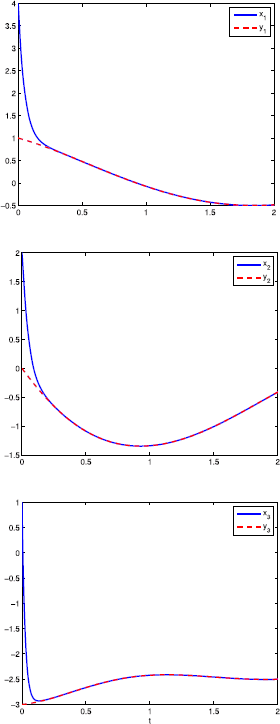Figure 3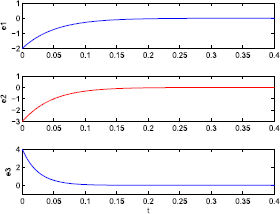Figure 4

To show the advantage of our applied exponential synchronization method using a nonlinear control function, the results are compared with synchronization based on the Lyapunov stability theorem. For the same system and initial conditions as shown in Figure 5, it is obvious that our methods have the advantage by compression synchronization times in Figure 3 of almost 0.1 second over approximately 4 second in Figure 5.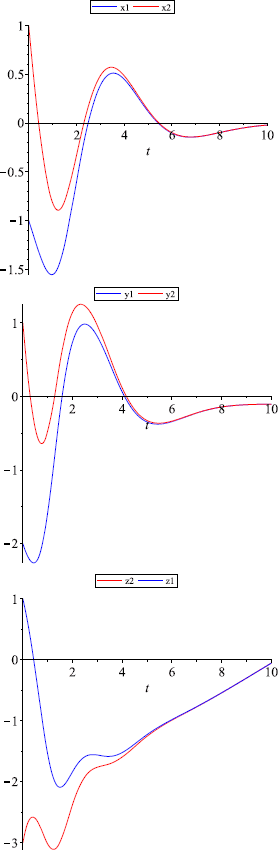Figure 5

The main benefit of the exponential synchronization method is the fast convergence. But there is a disadvantage in that, in practice, for some systems it is impossible or very difficult to obtain the exponential synchronization conditions. In this case, the exponential synchronization will change to synchronization based on the Lyapunov stability theorem. In simple terms, we can say exponential stability is Lyapunov stability, but the reverse is not true.

## Application of chaos to secure communication

Encryption of information is carried out when two persons want to communicate with each other by an insecure communicative channel that can be a telephone or network but the third person could not identify the exchanged information by listening to them. What the first person wants to send to the second person is called the context text; it can be an image or a text by any language that is completely optional. Before sending the message, the transmitter of information should encrypt the information by a specific key. In this case, the context text is converted into the encrypted text. The encrypted text passes through a communicative channel of two individuals and the second person could decrypt the text by using the key and the text is converted into a context text.

Our goal is to use chaotic systems for encryption. Since aperiodic waves of the chaotic systems cannot be predicted, these systems are important in secure communication. Also chaotic systems are sensitive to initial conditions, which is another advantage for using these systems in different applications of secure communication. Here encryption using modulation and the masking method is studied.

### Text modulation via adaptive exponential stability

In chaos modulation the information signal is injected into the transmitter. First we introduce some definitions, then we propose a fast mechanism for synchronization the chaotic systems without linear term (11) based on the drive-response approach. Using exponential synchronization, the secure communication scheme is studied.

### Definition 4.1



Consider the smooth system that is described by a variables vector $$X=\{x_{i}\}_{1}^{n}\in R^{1}$$ where the output vector is presented by $$Y=\{ y_{i}\}_{1}^{m}\in R^{1}$$:

$$\dot{x}=f(X,C),\qquad Y=h(X),$$
(29)

where the function $$h(\cdot)$$ is a smooth vector and the vector $$C\in R^{1}$$ is for constant parameters. Let $$Y^{i}$$, denote the jth time derivative of the vector Y. It is said that the vector state X is observable algebraically, if it is expressed uniquely by

$$X=\phi\bigl(Y,Y^{(1)},\ldots,Y^{(i)}\bigr),$$
(30)

for integer j and for smooth function ϕ.

### Definition 4.2



With the same conditions of Definition 4.2, if the vector of parameters, C, satisfies the following equation:

$$\psi_{1}\bigl(Y,Y^{(1)},\ldots,Y^{(i)}\bigr)= \psi_{2}\bigl(G,G^{(1)},\ldots,G^{(i)}\bigr)C,$$
(31)

where $$\psi_{1}(\cdot)$$ and $$\psi_{1}( \cdot)$$ are, respectively, $$n\times1$$ and $$n\times n$$ smooth parameters matrices, then C is said to be identifiable algebraically with respect to the output vector Y.

Now, assume the second differential equation of system (11) to be

$$x_{1}=\frac{\dot{y_{1}}}{z_{1}},$$
(32)

then substituting equation (32) into the third differential variables of system (11) leads to

$$\dot{z_{1}}z_{1}+\dot{y_{1}}y_{1}=bz_{1}.$$
(33)

According to Definitions 4.1 and 4.2 the system (11) is observable algebraically with respect to the two outputs $$y_{1}$$ and $$z_{1}$$. The parameter b in equations (33) and (11) is identifiable algebraically with respect to the two other available outputs. Therefore, the non-available state variable $$x_{1}$$ and the parameter b can be recovered simultaneously from the two other available outputs.

In the transmitter system, the parameter b is considered to transmit the message signal and the following drive system is defined:

\begin{aligned} & \dot{x_{1}}=\ln\bigl(a+e^{y_{1}-x_{1}}\bigr), \\ &\dot{y_{1}}=x_{1}z_{1}, \\ &\dot{z_{1}}=b(t)-x_{1}y_{1}, \end{aligned}
(34)

where $$x_{1}$$, $$y_{1}$$, $$z_{1}$$ are the state variables of chaotic system (11) with the modulation law

\begin{aligned} b(t)=o(t)+b, \end{aligned}
(35)

where $$o(t)$$ is the input message signal, which needs to be transmitted. In the receiver system, the response system is assumed to be the partly uncertain system (11) where the available outputs variables are $$y_{1}$$ and $$z_{1}$$. Hence, the response system is defined with controllers as follows:

\begin{aligned} &\dot{x_{2}}=\ln\bigl(a+e^{y_{1}-x_{2}} \bigr)+u_{1}, \\ &\dot{y_{2}}=x_{2}z_{1}+u_{2}, \\ &\dot{z_{2}}=\hat{b}(t)-x_{2}y_{1}+u_{3}, \end{aligned}
(36)

where $$x_{2}$$, $$y_{2}$$ and $$z_{2}$$ are the state variables of system (11) with the modulation laws

$$\hat{b}(t)=\hat{o}(t)+\hat{b},$$
(37)

where $$\hat{o}(t)$$ is the recovered signal and is the approximation of the unknown parameter b. $$U=[u_{1}, u_{2}, u_{3}]^{T}$$ is the input nonlinear control to achieve synchronization of the systems (34) and (36).

Subtracting the drive system (34) from the response system (36), the error system is obtained:

\begin{aligned} & \dot{e_{1}}=\ln\biggl(\frac{a+e^{-e_{1}}e^{y_{1}-x_{1}}}{a+e^{y_{1}-x_{1}}} \biggr)+u_{1}, \\ &\dot{e_{2}}=e_{1}z_{1}+u_{2}, \\ &\dot{e_{3}}=\tilde{o}{(t)}+\tilde{b}{(t)}-e_{1}y_{1}+u_{3}, \end{aligned}
(38)

where

$$\tilde{o}=\hat{o}-o, \qquad \tilde{b}=\hat{b}-b.$$
(39)

Therefore, for chaos synchronization, we need to define the controller U and a parameter approximation law to set the error system to converge to zero exponentially.

### Theorem 4.1

If the controller in the receiver system (36) is defined as follows:

\begin{aligned} &\dot{u_{1}}=-c-2\omega_{1}e_{1}, \\ &\dot{u_{2}}=-\omega_{2}e_{2}, \\ &\dot{u_{3}}=-\omega_{3}e_{3}, \end{aligned}
(40)

with the law parameter and message signal, respectively, chosen as

\begin{aligned} &\dot{\tilde{o}}=-e_{3}-\omega_{5}\tilde{o}, \\ &\dot{\tilde{b}}=-e_{3}-\omega_{4}\tilde{b}, \end{aligned}
(41)

and

\begin{aligned} &c>\ln\biggl(\frac{a+\operatorname{Exp}(-e_{1})\operatorname{Exp}(y_{1}-x_{1}}{a+\operatorname{Exp}(y_{1}-x_{1})}\biggr), \\ &\omega_{1}>\frac{1}{2}\biggl(\frac{k_{3}}{\rho_{1}}+ \frac{k_{2}}{\rho_{2}}\biggr), \\ &\omega_{2}>\frac{k_{3}}{2\rho_{1}}, \\ &\omega_{3}>\frac{k_{2}}{2\rho_{2}}, \\ &\omega_{4}>0, \\ &\omega_{5}>0, \end{aligned}
(42)

where $$\omega_{1}$$, $$\omega_{2}$$, $$\omega_{3}$$, $$\omega_{4}$$, $$\rho_{1}$$, $$\rho_{2}$$ are positive and $$| x_{i}|< k_{i}$$, $$i=1,2,3$$, then the response system (36) will synchronize the drive system (34) exponentially. Moreover, the message signal $$s(t)$$ will recover by the receiver system (36), which is combined into the chaotic transmitter (34) via the modulation laws (40) and (42).

### Proof

Assume $$E=[e_{1},e_{2},e_{3},\tilde{b},\tilde{o}]$$, by defining the quadratic Lyapunov function as

$$V(t)=EPE^{T}=\frac{1}{4}e^{2}_{1}+ \frac{1}{2}e^{2}_{2}+\frac{1}{2}e^{2}_{3}+ \frac {1}{2}\tilde{b}^{2}+\frac{1}{2}\tilde{o}^{2},$$
(43)

by the same proof as Theorem 3.3 exponentially stability can be achieved. □

To demonstrate and verify the validity of the modulation schemes, we confirmed our analytical studies be numerical simulations via MatLab software. We solve the dynamical systems using the fourth order Runge-Kutta numerical method. At the transmitter system side, the system parameters are chosen as $$a-0.1$$, $$b=0.25$$, while the arbitrary initial conditions are set as $$x_{1}(0)=1$$, $$x_{2}(0)=1$$, $$x_{3}(0)=-3$$. At the receiver system side, we fix the arbitrary initial conditions as $$y_{1}(0)=-1$$, $$y_{2}(0)=-2$$, $$y_{3}(0)=1$$, $$a=0.1$$, $$\hat{b}(0)=1$$ and $$\hat{o}(0)=0.8$$. Suppose the message signal $$o(t)$$ is a trigonometric signal such as

$$o(t)=0.05\sin(60\pi t).$$

Figure 6 displays the synchronization difference (error) between systems (34) and (36). It confirms that synchronized errors between system (34) and (36) converge exponentially to zero. Figure 7 shows the error signal between the original message and the recovered one that confirms the message signal $$o(t)$$ is recovered accurately. Figure 8 shows the value of the unknown parameters b which converge exponentially to the exact value.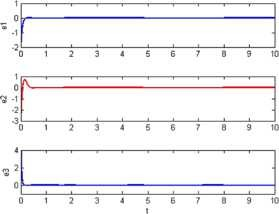Figure 6Figure 7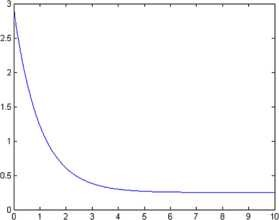Figure 8

### Modulation-based adaptive Lyapunov stability

Consider two drive and response systems, respectively, as drive system

\begin{aligned} \begin{aligned} &\dot{x_{1}}=\ln\bigl(a+e^{y_{1}-x_{1}}\bigr), \\ &\dot{y_{1}}=x_{1}z_{1}, \\ &\dot{z_{1}}=b-x_{1}y_{1}, \end{aligned} \end{aligned}
(44)

and

\begin{aligned} &\dot{x_{2}}=\ln\bigl(a+e^{y_{2}-x_{2}} \bigr)+u_{1}, \\ &\dot{y_{2}}=x_{2}z_{2}+u_{2}, \\ &\dot{z_{2}}=b-x_{2}y_{2}+u_{3}. \end{aligned}
(45)

We have introduced the three control functions $$u_{1}$$, $$u_{2}$$ and $$u_{3}$$ and define the error variables as

$$e_{1}=y_{1}-x_{1},\qquad e_{2}=y_{2}-x_{2},\qquad e_{3}=y_{3}-x_{3}.$$
(46)

Subtracting (44) from (45) and using the notation (46), we obtain the error system as

\begin{aligned} &\dot{e_{1}} = \ln\bigl(a+e^{y_{2}-x_{2}} \bigr) -\ln\bigl(a+e^{y_{1}-x_{1}}\bigr)+u_{1} , \\ &\dot{e_{2}} = x_{2} z_{2} - x_{1} z_{1}+ u_{2} , \\ &\dot{e_{3}} = -y_{2}x_{2}+y_{1} x_{1} + u_{3} . \end{aligned}
(47)

### Theorem 4.2

For any initial conditions, if the controller functions $$u_{1}$$, $$u_{2}$$ and $$u_{3}$$ are designed with

\begin{aligned} &u_{1} = \ln\biggl(\frac{a+e^{y_{1}-x_{1}}}{a+e^{y_{2}-x_{2}}}\biggr) - e_{1}, \\ &u_{2} = e_{3} e_{1} + e_{1} z_{1}+ x_{1} e_{3}-e_{2}, \\ &u_{3} = -e_{1} e_{2} -e_{2}x_{1} -e_{1} y_{1} - e_{3}, \end{aligned}
(48)

and the updating laws of the unknown parameters are chosen by

$$\dot{\hat{a}}(t) = \tilde{a}_{1}, \qquad \dot{\hat{b}}(t) = \tilde{b}_{1},$$
(49)

where $$\hat{a}=a-\tilde{a}$$ and $$\hat{ b}=b-\tilde{b}$$ are the estimates of the unknown parameters, a and b, then the trajectory of the error system converge to zero exponentially.

### Proof

If the positive Lyapunov function is chosen as

$$V= \frac{1}{2}\bigl(e^{2}_{1}+e^{2}_{2}+ \tilde{a}^{2}+\tilde{b}^{2}\bigr),$$

with the choice of the controller (48) and updating laws (49), then the time derivative of V along the trajectory of the system (44) and (45) is negative definite. So, according to the Lyapunov theorem, the synchronization of systems (44) and (45) is achieved under the chosen controller u and parameters updating laws. □

To demonstrate and verify the validity of the modulation schemes, we confirmed our analytical studies be numerical simulations via MatLab software. We solve the dynamical systems using the fourth order Runge-Kutta numerical method. At the transmitter system side, the system parameters are chosen as $$a=0.1$$, $$b=0.25$$ while the arbitrary initial conditions are set as $$x_{1}(0)=1$$, $$y_{1}(0)=1$$, $$z_{1}(0)=-3$$. At the receiver system side, we fix the arbitrary initial conditions as $$x_{2}(0)=-1$$, $$y_{2}(0)=-2$$, $$z_{2}(0)=1$$, $$a=0.1$$, $$\hat{b}(0)=1$$ and $$\hat{o}(0)=0.8$$. Suppose the message signal $$o(t)$$ is trigonometric signal such as $$o(t)=0.05\sin(60\pi t)$$, which is added on the second state variable of the drive system.

Figure 9 displays the synchronization difference (error) between systems (44) and (45). Figure 10 shows the error signal between the original message and the recovered one that confirms that the message signal $$o(t)$$ is recovered accurately. Figure 11 shows the value of the unknown parameters a, b and the error between sent and received signal.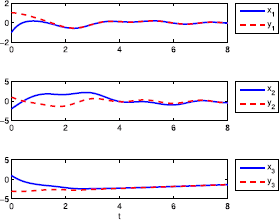Figure 9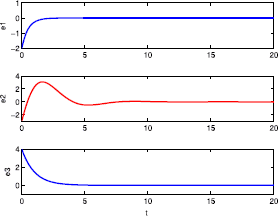Figure 10Figure 11

By compression of results, it is obvious that in exponential synchronization the error system very fast tends to zero, which results in sending and receiving the signal faster with a better accuracy.

### Image encryption using exponential stability

Almost in all cases the image encryption is often conducted along with data compression before be saved or transmitted. This is because of high volume of pictorial data and their adjuncts. Thus, it is so important to combine security demands with compression systems. In view of security, the pictorial data is not sensitive the same as the context data. In block method of encryption, firstly, one encryption key with equal dimensions with designed images one is produced by using of chaotic system and then the primary image encoded by block cipher.

Here, we have only one block. The given method could be exercised by producing keys with small sizes in a higher block number.

In order to decode, the encrypted image is produced by applying the same chaotic system and utilization of an equal initial value and by considering inversion methods in the decoding key.

In the masking method for image encryption, the information signal is combined linearly and added to the drive system. Suppose $$o(t)$$ is the original image, the transmitted message $$s(t)$$ is assumed as

$$s(t)=o(t)+k_{1}x_{1}(t)+k_{2}y_{1}(t)+k_{3}z_{1}(t),$$
(50)

where $$k_{i}>0$$, $$i=1,2,3$$ and $$x_{1}$$, $$y_{1}$$, $$z_{1}$$ are the state variables. The encrypted signal is masked using an arbitrary function of master chaotic system variables to send and receive via a public channel. The assumed $$T_{s}$$ is the synchronization time and the image is added after that time. The recovered image $$r(t)$$ is obtained using the following relation:

$$r(t)=s(t)-k_{1}x_{2}(t)-k_{2}y_{2}(t)-k_{3}z_{2}(t).$$
(51)

Because

\begin{aligned} r(t) =&s(t)-k_{1}x_{2}-k_{2}y_{2}-k_{3}z_{2} \\ =&o(t)+k_{1}x_{1}+k_{2}y_{1}+k_{3}z_{1}-k_{1}x_{2}-k-2y-2-k_{3}z_{2} \\ =&o(t)+k_{1}e_{1}+k_{2}e_{2}+k_{3}e_{3} \\ \cong& o(t). \end{aligned}
(52)

That obviously depends on the synchronization time.

To show the validity of the proposed method, simulation results using Matlab software and the Runge-Kutta method are applied for the initial condition $$(x_{1}(0),y_{1}(0),z_{1}(0))=(1,-1,2)$$ and $$(x_{2}(0),y_{2}(0),z_{2}(0))=(3,1,-1)$$ on the system (11) without linear term and a digital image as a signal in Figure 12, Figure 13 and Figure 14.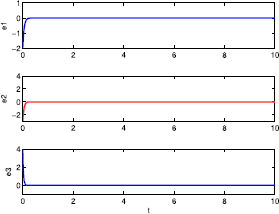Figure 12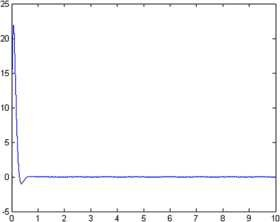Figure 13Figure 14

## Conclusion

In this paper, we showed that exponential stability method for the synchronization of chaotic dynamical systems is faster than of the Lyapunov stability theorem which it confirmed by the results obtained for a system without linear term. By using presented synchronization, cryptography of text and image were given through both modulation and masking methods in both active and adaptive cases. The results confirm the rate and precision of reception and transmittance of the desired signal.

Further research topics include the applying optimal control in the exponential synchronization of classical and fractional order chaotic systems and study of the other encryption methods such as the Rivest-Shamir-Adlemen (RSA) and Data Encryption Standard (DES) to compare them with the chaos-based encryption.

## References

1. 1.

Lorenz, EN: Deterministic nonperiodic flow. J. Atmos. Sci. 20(2), 130-141 (1963)

2. 2.

Ott, E, Grebogi, C, Yorke, JA: Controlling chaos. Phys. Rev. Lett. 64(11), 1196 (1990)

3. 3.

Pecora, LM, Carroll, TL: Synchronization in chaotic systems. Phys. Rev. Lett. 64(8), 821 (1990)

4. 4.

Yu, S, Lu, J, Leung, H, Chen, G: Design and implementation of n-scroll chaotic attractors from a general jerk circuit. IEEE Trans. Circuits Syst. I, Regul. Pap. 52(7), 1459-1476 (2005)

5. 5.

Yu, F, Wang, C: Secure communication based on a four-wing chaotic system subject to disturbance inputs. Optik 125(20), 5920-5925 (2014)

6. 6.

Grassi, G, Mascolo, S: Synchronizing high dimensional chaotic systems via eigenvalue placement with application to cellular neural networks. Int. J. Bifurc. Chaos 9(4), 705-711 (1999)

7. 7.

Hsieh, J-Y, Hwang, C-C, Wang, A-P, Li, W-J: Controlling hyperchaos of the Rossler system. Int. J. Control 72(10), 882-886 (1999)

8. 8.

Kapitaniak, T, Chua, LO, Zhong, G-Q: Experimental hyperchaos in coupled Chua’s circuits. IEEE Trans. Circuits Syst. I, Fundam. Theory Appl. 41(7), 499-503 (1994)

9. 9.

Peng, JH, Ding, EJ, Ding, M, Yang, W: Synchronizing hyperchaos with a scalar transmitted signal. Phys. Rev. Lett. 76(6), 904 (1996)

10. 10.

Vicente, R, Daudén, J, Colet, P, Toral, R: Analysis and characterization of the hyperchaos generated by a semiconductor laser subject to a delayed feedback loop. IEEE J. Quantum Electron. 41(4), 541-548 (2005)

11. 11.

Posadas-Castillo, C, López-Gutiérrez, RM, Cruz-Hernández, C: Synchronization of chaotic solid-state Nd:YAG lasers: application to secure communication. Commun. Nonlinear Sci. Numer. Simul. 13(8), 1655-1667 (2008)

12. 12.

Park, JH: Chaos synchronization of a chaotic system via nonlinear control. Chaos Solitons Fractals 25(3), 579-584 (2005)

13. 13.

Agiza, HN, Yassen, MT: Synchronization of Rossler and Chen chaotic dynamical systems using active control. Phys. Lett. A 278(4), 191-197 (2001)

14. 14.

Aghababa, MP: Robust stabilization and synchronization of a class of fractional-order chaotic systems via a novel fractional sliding mode controller. Commun. Nonlinear Sci. Numer. Simul. 17(6), 2670-2681 (2012)

15. 15.

Aghababa, MP, Aghababa, HP: Synchronization of nonlinear chaotic electromechanical gyrostat systems with uncertainties. Nonlinear Dyn. 67(4), 2689-2701 (2012)

16. 16.

Mossa Al-Sawalha, M, Salmi Md Noorani, M: Anti-synchronization of two hyperchaotic systems via nonlinear control. Commun. Nonlinear Sci. Numer. Simul. 14(8), 3402-3411 (2009)

17. 17.

Yang, C-C: One input control of exponential synchronization for a four-dimensional chaotic system. Appl. Math. Comput. 219(10), 5152-5161 (2013)

18. 18.

Liao, X, Yu, P: Study of globally exponential synchronization for the family of Rössler systems. Int. J. Bifurc. Chaos 16(8), 2395-2406 (2006)

19. 19.

Wang, Z: Anti-synchronization in two non-identical hyperchaotic systems with known or unknown parameters. Commun. Nonlinear Sci. Numer. Simul. 14(5), 2366-2372 (2009)

20. 20.

Tong, D, Zhu, Q, Zhou, W, Xu, Y, Fang, J: Adaptive synchronization for stochastic T-S fuzzy neural networks with time-delay and Markovian jumping parameters. Neurocomputing 117, 91-97 (2013)

21. 21.

Tong, D, Zhang, L, Zhou, W, Zhou, J, Xu, Y: Asymptotical synchronization for delayed stochastic neural networks with uncertainty via adaptive control. Int. J. Control. Autom. Syst. 14(3), 706-712 (2016)

22. 22.

Tong, D, Zhou, W, Zhou, X, Yang, J, Zhang, L, Xu, Y: Exponential synchronization for stochastic neural networks with multi-delayed and Markovian switching via adaptive feedback control. Commun. Nonlinear Sci. Numer. Simul. 29(1), 359-371 (2015)

23. 23.

Yan, Z, Yu, P: Globally exponential hyperchaos (lag) synchronization in a family of modified hyperchaotic Rössler systems. Int. J. Bifurc. Chaos 17(5), 1759-1774 (2007)

24. 24.

Cuomo, KM, Oppenheim, AV, Strogatz, SH: Synchronization of Lorenz-based chaotic circuits with applications to communications. IEEE Trans. Circuits Syst. II, Analog Digit. Signal Process. 40(10), 626-633 (1993)

25. 25.

Boutayeb, M, Darouach, M, Rafaralahy, H: Generalized state-space observers for chaotic synchronization and secure communication. IEEE Trans. Circuits Syst. I, Fundam. Theory Appl. 49(3), 345-349 (2002)

26. 26.

Stevens, BL, Lewis, FL, Johnson, EN: Aircraft Control and Simulation: Dynamics, Controls Design, and Autonomous Systems. Wiley, New York (2015)

27. 27.

Teschl, G: Ordinary Differential Equations and Dynamical Systems. Graduate Studies in Mathematics, vol. 140. Am. Math. Soc., Providence (2012)

28. 28.

Narendra, KS, Annaswamy, AM: Stable Adaptive Systems. Dover, Mineola (2012)

29. 29.

Khalil, HK: Nonlinear Systems, 3rd edn. Pearson, Harlow (2002)

30. 30.

Shankar, S, Bodson, M: Adaptive Control: Stability, Convergence and Robustness. Dover, Mineola (2011)

31. 31.

Aghababa, MP, Khanmohammadi, S, Alizadeh, G: Finite-time synchronization of two different chaotic systems with unknown parameters via sliding mode technique. Appl. Math. Model. 35(6), 3080-3091 (2011)

32. 32.

Xu, Y, Wang, Y: A new chaotic system without linear term and its impulsive synchronization. Optik 125(11), 2526-2530 (2014)

33. 33.

Mata-Machuca, JL, Martínez-Guerra, R, Aguilar-López, R, Aguilar-Ibañez, C: A chaotic system in synchronization and secure communications. Commun. Nonlinear Sci. Numer. Simul. 17(4), 1706-1713 (2012)

Download references

## Author information

Authors

### Corresponding author

Correspondence to Mahmoud Maheri.

## Additional information

### Competing interests

The authors declare that they have no competing interests.

### Authors’ contributions

All authors jointly worked on deriving the results and approved the final manuscript.

### Publisher’s Note

Springer Nature remains neutral with regard to jurisdictional claims in published maps and institutional affiliations.

## Rights and permissions

Open Access This article is distributed under the terms of the Creative Commons Attribution 4.0 International License (http://creativecommons.org/licenses/by/4.0/), which permits unrestricted use, distribution, and reproduction in any medium, provided you give appropriate credit to the original author(s) and the source, provide a link to the Creative Commons license, and indicate if changes were made.

Reprints and Permissions